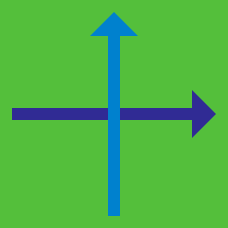Geometry

# Locus of Points

Given $A=(5,0)$ and $B=(0,4)$, what is the equation of the locus of points $P$ such that $\lvert \overline{PA} \rvert^2- \lvert \overline{PB} \rvert^2=9?$

Given $A=(9,-1)$ and $B=(-9,5)$, what is the equation of the locus of points $P$ such that $\lvert \overline{AP} \rvert: \lvert \overline{BP} \rvert=1:2 ,$ where $\lvert \overline{AP} \rvert$ denotes the length of $\overline{AP}?$

Let $A=(6,-5)$ and $B=(-6,1)$ be two vertices of triangle $ABC.$ What is the locus of vertex $C$ such that the centroid of $\triangle ABC$ moves along the line $2x+5y=1?$

Let $A=(-6,0)$, $B=(3,-3)$ and $C=(5,3)$. What is the equation of the locus of points $P$ such that $\lvert \overline{AP} \rvert^2+\lvert \overline{BP} \rvert^2=2 \lvert \overline{CP} \rvert^2?$

If $A=(-3,0)$ and $B=(12,0),$ what is the equation for the locus of points $P$ such that $\lvert \overline{PA} \rvert =\lvert \overline{PB} \rvert,$ where $\lvert \overline{PA} \rvert$ denotes the length of $\overline{PA}?$

×